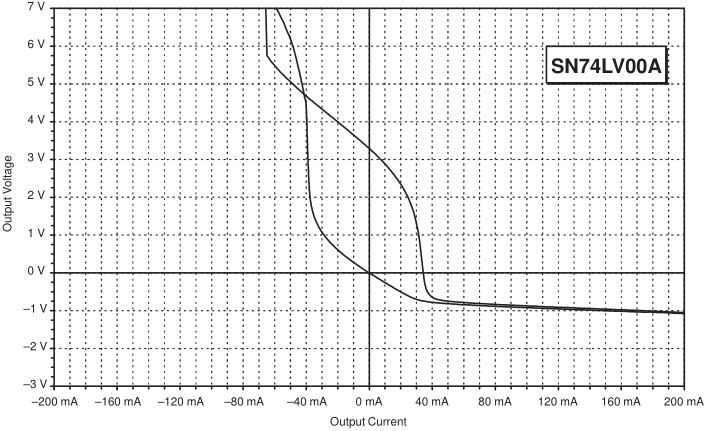If you have a related question, please click the "Ask a related question" button in the top right corner. The newly created question will be automatically linked to this question.

# SN74LV123A: Output Voltage vs. Current

Part Number: SN74LV123A

Hi All,

Customer is using the SN74LV123A to drive the LED of an optocoupler with 100 ohm resistor in series.

The SPICE simulation shows that the output drops (1Q) to ~1.5V with 2.7 mA being drawn, Vdd is 3.3V and ~ 1.4V dropped by the LED.  This appears to make sense 1.4V + 100*2.7mA is ~ 1.7V which leaves 3.3V-1.7V at the output of the SN74LV123A (1Q).

There is no information in the datasheet mentioning the output voltage versus current.

1. Is the simulation model correct? Will the output voltage drop to 1.5 V if the device output is loaded in the described manner?
1. Is it ok to draw this that much current from the part? Will the part be damage in the long run.

Thanks,

Regards,

Joe

• You are not allowed to exceed the absolute maximum rating (25 mA).

The electrical characteristics are guaranteed only for up to 6 mA at 3 V. If you exceed this, the voltage drop over the output transistor might become much larger.

So 2.7 mA is fine.

The voltage drop should be much smaller.• Hey Joe,

Which simulation model is being used, and in which simulation software is it being used?

a. Is the simulation model correct? Will the output voltage drop to 1.5 V if the device output is loaded in the described manner?

No, the simulation is not correct. The output will be much closer to 3.3V.  We can approximate the internal resistance of the output (worst case) from the datasheet values for VOH and IOH:

Rp = (3 - 2.48)/0.006 = 86.67 ohms

At 3.3V, we can easily calculate the output voltage with your given load from KVL and Ohm's Law (assuming the diode drops 1.4V):

First the current output:  (3.3 - 1.4)/(100+86.67) = 10.18 mA

Then the voltage drop across the internal resistance (pFET):  0.01018 * 86.67 = 0.882 V

So, the worst case VOH would be approximately 3.3 - 0.882 = 2.418 V

Under normal circumstances (typical device at room temp), I would expect to see about half that resistance (40 ohms), so about 2.75 V at the output.

a. Is it ok to draw this that much current from the part? Will the part be damage in the long run.

Yes, you are well below the rated maximum for the device. The 6mA rating is only to indicate the output voltage test value -- it is not a limitation.  The correct limiting factor is what Clemens mentioned, which is 25 mA (per output, 50mA total).

• For anyone who finds this thread in the future - the model being used was the PSpice model available on TI.com which is limited to only 5V operation - at 3V it has erroneous results.

The customer's bench tests confirmed my estimation of 2.75V at the output of the real device.